# Calculating moving average in Excel

In this short tutorial, you will learn how to quickly calculate a simple moving average in Excel, what functions to use to get moving average for the last N days, weeks, months or years, and how to add a moving average trendline to an Excel chart.

In a couple of recent articles, we have taken a close look at calculating average in Excel. If you've been following our blog, you already know how to calculate a normal average and what functions to use to find weighted average. In today's tutorial, we will discuss two basic techniques to calculate moving average in Excel.

## What is moving average?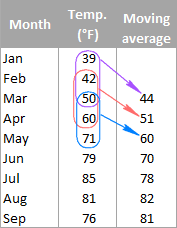Generally speaking, moving average (also referred to as rolling average, running average or moving mean) can be defined as a series of averages for different subsets of the same data set.

It is frequently used in statistics, seasonally-adjusted economic and weather forecasting to understand underlying trends. In stock trading, moving average is an indicator that shows the average value of a security over a given period of time. In business, it's a common practice to calculate a moving average of sales for the last 3 months to determine the recent trend.

For example, the moving average of three-month temperatures can be calculated by taking the average of temperatures from January to March, then the average of temperatures from February to April, then of March to May, and so on.

There exist different types of moving average such as simple (also known as arithmetic), exponential, variable, triangular, and weighted. In this tutorial, we will be looking into the most commonly used simple moving average.

## Calculating simple moving average in Excel

Overall, there are two ways to get a simple moving average in Excel - by using formulas and trendline options. The following examples demonstrate both techniques.

### Calculate moving average for a certain time period

A simple moving average can be calculated in no time with the AVERAGE function. Supposing you have a list of average monthly temperatures in column B, and you want to find a moving average for 3 months (as shown in the image above).

Write a usual AVERAGE formula for the first 3 values and input it in the row corresponding to the 3rd value from the top (cell C4 in this example), and then copy the formula down to other cells in the column:

`=AVERAGE(B2:B4)`

You can fix the column with an absolute reference (like \$B2) if you want to, but be sure to use relative row references (without the \$ sign) so that the formula adjusts properly for other cells.

Remembering that an average is computed by adding up values and then dividing the sum by the number of values to be averaged, you can verify the result by using the SUM formula:

`=SUM(B2:B4)/3`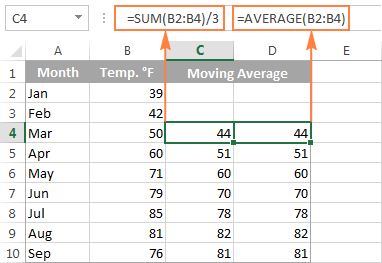### Get moving average for a the last N days / weeks / months/ years in a column

Supposing you have a list of data, e.g. sale figures or stock quotes, and you want to know the average of the last 3 months at any point of time. For this, you need a formula that will recalculate the average as soon as you enter a value for the next month. What Excel function is capable of doing this? The good old AVERAGE in combination with OFFSET and COUNT.

=AVERAGE(OFFSET(first cell, COUNT(entire range)-N,0,N,1))

Where N is the number of the last days / weeks / months/ years to include in the average.

Not sure how to use this moving average formula in your Excel worksheets? The following example will make things clearer.

Assuming that the values to average are in column B beginning in row 2, the formula would be as follows:

`=AVERAGE(OFFSET(B2,COUNT(B2:B100)-3,0,3,1))`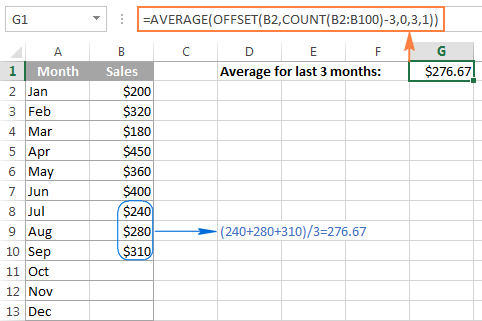And now, let's try to understand what this Excel moving average formula is actually doing.

• The COUNT function COUNT(B2:B100) counts how many values are already entered in column B. We start counting in B2 because row 1 is the column header.
• The OFFSET function takes cell B2 (the 1st argument) as the starting point, and offsets the count (the value returned by the COUNT function) by moving 3 rows up (-3 in the 2nd argument). As the result, it returns the sum of values in a range consisting of 3 rows (3 in the 4th argument) and 1 column (1 in the last argument), which is the latest 3 months that we want.
• Finally, the returned sum is passed to the AVERAGE function to calculate the moving average.

Tip. If you are working with continuously updatable worksheets where new rows are likely to be added in the future, be sure to supply a sufficient number of rows to the COUNT function to accommodate potential new entries. It's not a problem if you include more rows than actually needed as long as you have the first cell right, the COUNT function will discard all empty rows anyway.

As you probably noticed, the table in this example contains data for only 12 months, and yet the range B2:B100 is supplied to COUNT, just to be on the save side :)

### Find moving average for the last N values in a row

If you want to calculate a moving average for the last N days, months, years, etc. in the same row, you can adjust the Offset formula in this way:

=AVERAGE(OFFSET(first cell,0,COUNT(range)-N,1,N,))

Supposing B2 is the first number in the row, and you want to include the last 3 numbers in the average, the formula takes the following shape:

`=AVERAGE(OFFSET(B2,0,COUNT(B2:N2)-3,1,3))`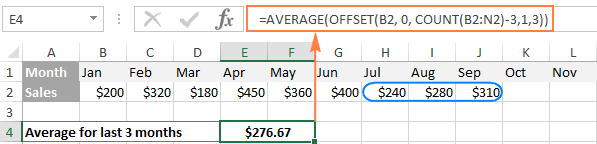## Creating an Excel moving average chart

If you have already created a chart for your data, adding a moving average trendline for that chart is a matter of seconds. For this, we are going to use Excel Trendline feature and the detailed steps follow below.

For this example, I've created a 2-D column chart (Insert tab > Charts group) for our sales data: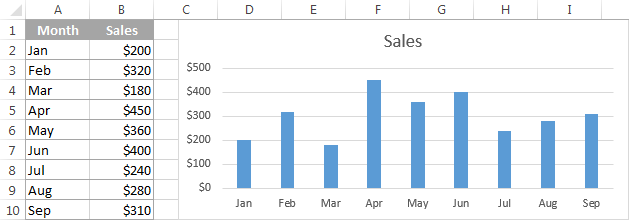And now, we want to "visualize" the moving average for 3 months.

1. In Excel 2013, select the chart, go to the Design tab > Chart Layouts group, and click Add Chart Element > Trendline > More Trendline Options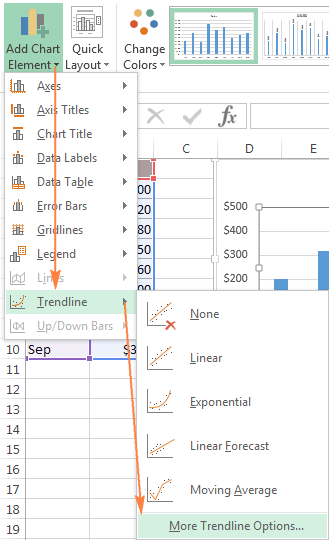In Excel 2010 and Excel 2007, go to Layout > Trendline > More Trendline Options.

Tip. If you do not need to specify the details such as the moving average interval or names, you can click Design > Add Chart Element > Trendline > Moving Average for the immediate result.

2. The Format Trendline pane will open on the right-hand side of your worksheet in Excel 2013, and the corresponding dialog box will pop up in Excel 2010 and 2007.

On the Format Trendline pane, you click the Trendline Options icon, select the Moving Average option and specify the moving average interval in the Period box: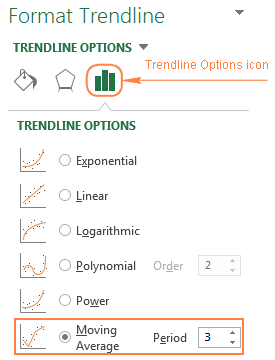3. Close the Trendline pane and you will find the moving average trendline added to your chart: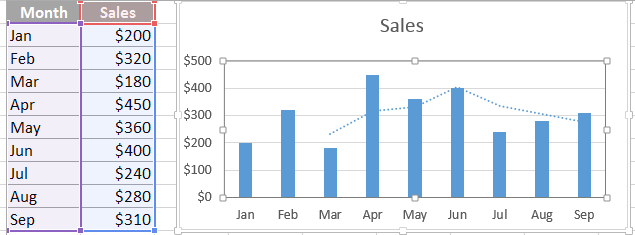To refine your chat, you can switch to the Fill & Line or Effects tab on the Format Trendline pane and play with different options such as line type, color, width, etc.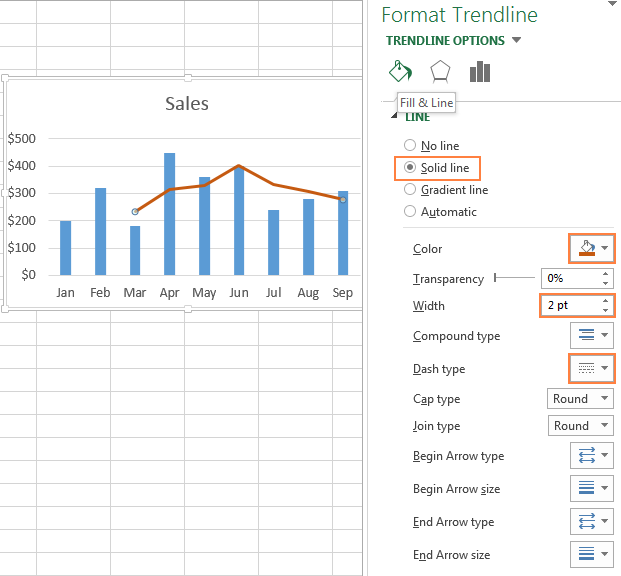For powerful data analysis, you may want to add a few moving average trendlines with different time intervals to see how the trend evolves. The following screenshot shows the 2-month (green) and 3-month (brick red) moving average trendlines: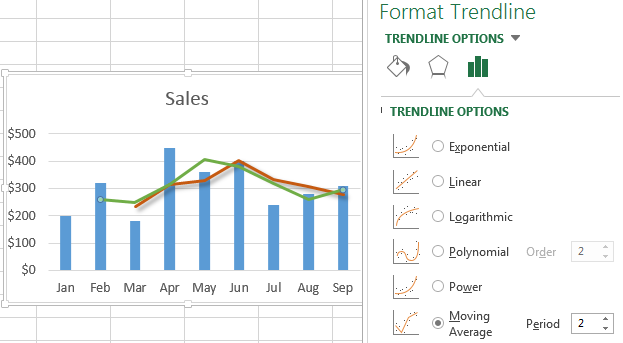Well, that's all about calculating moving average in Excel. The sample worksheet with the moving average formulas and trendline is available for download at the end of this post. I thank you for reading and look forward to seeing you next week!

## Practice workbook

Calculating moving average - examples (.xlsx file)

## You may also be interested in

1. Hi Alex,

How would I go about this in the reverse? Ie; What I need my average sale to be to reach my budget?

As an example; my budget is 20,000. I have gotten 15 sales so far in the year for 8,000 total, and expecting another 15 sales for the rest of the year. I would need an average of 800 per sale to reach my budget.

But how do I put this in a formula so it will show the average sale required to reach my budget (or goal in this case)?

Thank you :)

• This is a task for the school: (20000-8000)/15

2. Can we do this same when performing weighted moving average? If yes, how to go about it. Thanks.

X , Y

8 , 6
8.1 , 3.3
8.2 , 1
8.3 , 0.3
8.4 , 0.2

for all remaining values

Thanks and Regards

Sushant

4. X Y
8 6
8.1 3.3
8.2 1
8.3 0.3
8.4 0.2
8.5 1.1
8.6 5.2
8.7 10.4
8.8 18
8.9 28.9
9 35
9.1 42.9
9.2 44.1
9.3 43.2
9.4 40.5
9.5 35.8
9.6 28.1
9.7 23.1
9.8 19
9.9 16.1
10 11

I want to find X values for every Y=0.4 value

5. Hi Svetlana,

XY scatter plot of my data gives a sinusoidal chart. Now I want to calculate X unknown values for some particular Y values. Means I want moving average equation which will provide me with X values for my chosen Y values

6. I'm looking to calculate a rolling percentage of A/R amounts split out by date range. So I have my A/R aging in rows 17-21, and 22 autosums them, and each column is labeled with a different month. I need a formula to get a cell's percentage of the total for the last month only. Thanks.

7. How to find each product wise average of last 30 rows / values.

Thanks.....

8. This is in reference to: "Find moving average for the last N values in a row"

How would the formula change if you are trying to find the MA for the FIRST N values instead of the last? I have my data set up in reverse chronological order for a scorecard.

Thanks!

• I tried a different approach. Went to the "Define Name" part of the Formulas tab and named cells F6:R6 as "Rolling_Average" and then I entered =Average(Rolling_Average) in cell E6. The Rolling average forumla seems to average F6:R6 regardless of how many columns I add.

9. Hi
Super tutorial. Thanks.
Can anyone help with this?
I'm trying 7 day rolling average for an entire column (A:A) which gets added to everyday (blanks at the end of column ignored) and output that result to an assigned cell. That's explained perfectly in the tutorial above.
But how do I calculate rolling average for entire column entries except the last entry and this result returned to another assigned cell?

I.E - if I have 100 days data in a column. I want rolling average from days 94-100 returned to a particular cell. I also want rolling average for days 93-99 returned to another cell and rolling average for days 92-98 returned to a 3rd cell?

Thanks
D

• Hi!
With the OFFSET function, you can get the range of values you need.

=OFFSET(B1,COUNT(B1:B100)-8,0,7,1)
=OFFSET(B1,COUNT(B1:B100)-9,0,7,1)
=AVERAGE( OFFSET(B1,COUNT(B1:B100)-9,0,7,1) )

10. Great work
Keep it up

11. I have planned to provide X orders during 12 months and for this purpose I calculated average (12 months) but in certain months the orders are differ from average planned amounts, so I would like to make another average with rest of planned orders for the rest period? What formula can I apply. Thank you in advance

12. Hi.

Anyone who can help me out with my excel data? I would need a graph in excel that would show how many orders were created in 7 days rolling period. Is this possible? Any help would be much appreciated. Thank you

13. This was exactly what I needed! I was running trimmean and wanted it for the last 30 records. I was manually adjusting my formula range every time I added a record. ugh!

Thanks!

14. How do I Calculate last three months new joiners avg?

• Hello!
I’m sorry but your task is not entirely clear to me. For me to be able to help you better, please describe your task in more detail. Please specify what you were trying to find, what formula you used and what problem or error occurred. Give an example of the source data and the expected result.
It’ll help me understand it better and find a solution for you. Thank you.

15. Hello,
I have workbooks where each book represents a year of data.
Within each workbook I have daily data entered. I have a summary page in each book that displays current month average, last 3 months average and last 6 months average.
Here is the dilemma.
Say it is January, so a new book. For last 3 months I need to be able to pull the last two months of the previous book but come February, of course it needs only December from the previous book and similarly for the 6 months rolling average. I currently am using the below formula for my rolling average which ignores the first two months and does not generate an average till after there is March data. But I want a true rolling three months ( and 6 months ) average. How should I proceed?
Thank you
for 3 month's rolling average:
=IF(COUNT(M5:M16)<3,"n/a",AVERAGE(OFFSET(M5,COUNT(M5:M16)-3,0,3,1)))
for 6 month's rolling average:
=IF(COUNT(M5:M16)<6,"n/a",AVERAGE(OFFSET(M5,COUNT(M5:M16)-6,0,6,1)))

• Hello!
Unfortunately, without seeing your data it is impossible to give you advice.

I'm sorry, it is not very clear what result you want to get. Could you please describe your task in more detail and send us a small sample workbook with the source data and expected result to support@ablebits.com? Please shorten your tables to 10-20 rows/columns and include the link to your blog comment.

16. Hi Svetlana and everyone :)
I have data ranges of over 100 cells. Let us say A1:A100, as an example. I need to find the maximum cumulative average from within that range (A1:A100) that is at least 6o cells long. To put it differently, the cumulative average has to have at least 60 entries to qualify as the maximum.

Would be fascinated to know if there is a formula(s) to solve this.

Thank you

Robert

17. what is the formula to average a column (in the adjacent column) each time a new (entry) row is added at the bottom? in other words, the first cell of the average is always the top cell in the original column, but the last cell to be included in the average is the new row.

• Hello!
If I understand you correctly, you want to calculate the average in one cell. The AVERAGE function ignores empty cells. Therefore, you can calculate the average over a large data range to which you will add new data.
For example, = AVERAGE (F2: F1000)

If my guess is not correct, then use the instruction from Example 1 above.

18. Hi,
I have data starting from A5 to A2000 (repetitive I.Ds) and B5 to B2000 (£value of each I.d).
Now, I have a mechanism that as soon as I Enter an ID in A4, it only fetch the £values related to this ID in A4 and all other IDs and £values will remain hidden.
Now, I only want to take the average of last 5 £values for the selected ID and ignore the rest hidden £values as well as the hidden rows,...
I have used AverageIfs, subtotal As well as many other ways with the offset to get the job done but no luck at all.
I appreciate it if you could help me with the above scenario. Many thanks